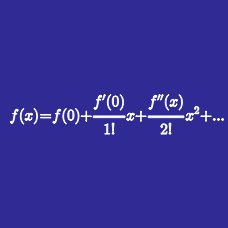Calculus

# Taylor Series

Determine the Taylor series for the function $f(x) = \sin(x)\cos(x) \text{ centered at } x = \pi.$

At $a = 0$, what is the Taylor series expansion of

$\ln ( 1 + x ) ?$

At $a = 0$, what is the Taylor series expansion of

$\frac{1}{(1-x)^2} ?$

Given the Maclaurin series expansion of $\exp(x^2)$ as $a_0 + a_1 x^1 + a_2 x^2 + \cdots ,$ what is the value of $a_0 + a_1 + a_2$?

Determine the first three non-zero terms of the Taylor series for $f(x) = \tan(x) \text{ centered at } x = \frac{\pi}{4}.$

×